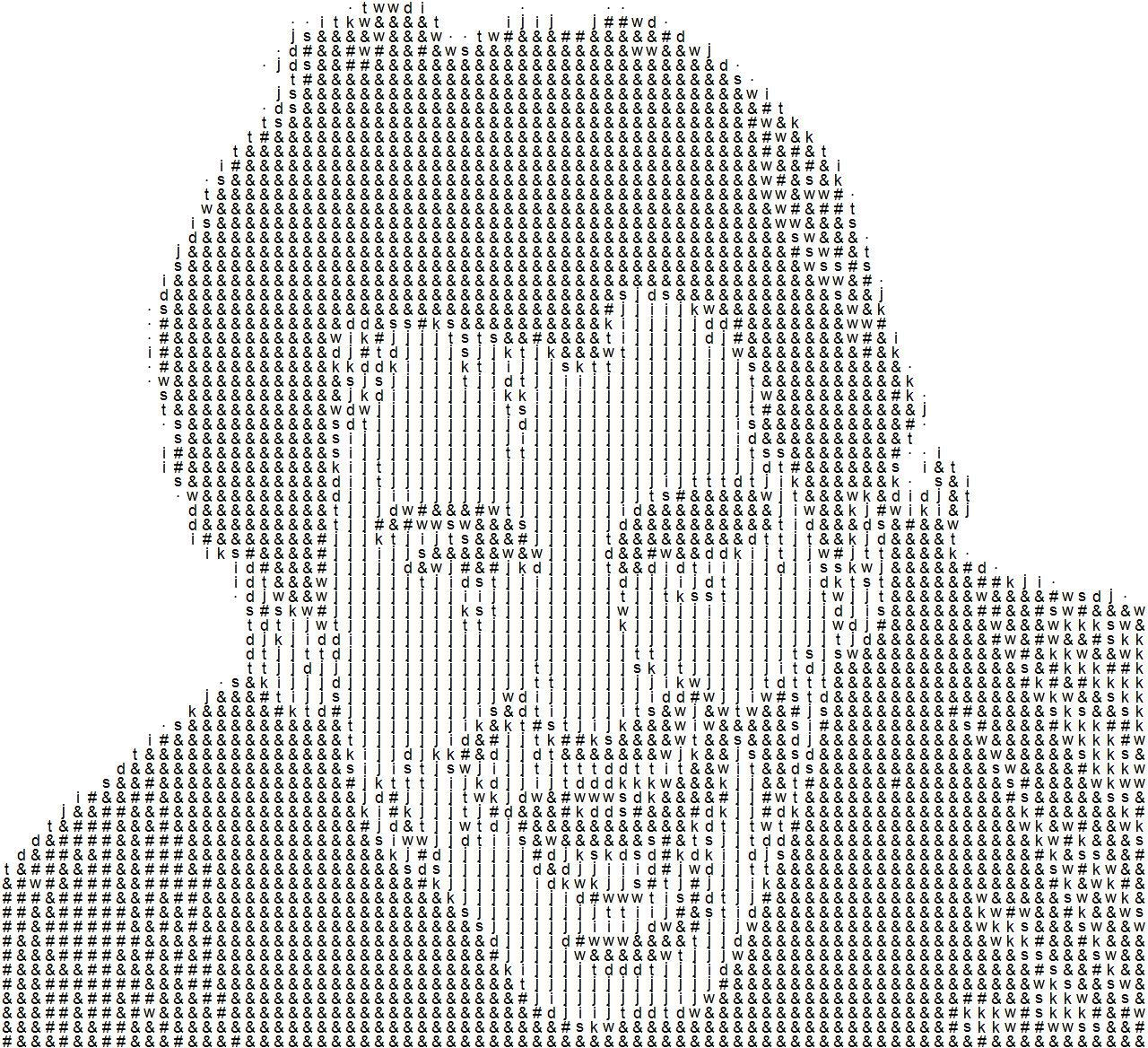## 1. 基本原理

• 将彩色图片转化为灰度图(像素值从0到255，其中0为黑色，255为白色)
• 设计一套字符集来对应各像素值，一般原则是：黑色对应最复杂字符(比如`&``@`)；白色对应最简单字符(比如`.`或空格)
• 根据彩色图片的RGB各通道的像素值来给字符上色
RGB色彩模式是通过对红(R)、绿(G)、蓝(B)三个颜色通道的叠加来得到各式各样的颜色的，常用的是8位图(各通道均为256等级，数值从0, 1, 2, ...直到255)。灰度图只含一个通道，不含色彩信息，就是我们平时看到的黑白照片，通常也划分为0到255共256个级别，其中0最暗(全黑)，255最亮(全白)。在ImageMagick中，从彩色图片中的RGB值到灰度值Gray转换公式默认为：`Gray = 0.212656*R + 0.715158*G + 0.072186*B`

``````[0, 23) → '&'
[23, 46) → '#'
[46, 69) → 'w'
......
......
[209, 232) → '.'
[232, 255] → ' '``````

## 2. R实现

``````library(magick)  # 加载magick包

image2chars <- function(pathIn='',
pathOutTxt=NULL,
pathOutImg=NULL,
jpg_quality=80,
width=100,
chars=c('&','#','w','s','k','d','t','j','i','.', ' '),
isColor=FALSE){
##### 参数
# pathIn: 原始图片的路径，支持各种格式
# pathOutTxt: 字符文本的输出路径，默认与原始图片在同一文件夹下，只是后缀为.txt;你也可指定其他路径
# pathOutImg: 字符图片的输出路径，默认与原始图片在同一文件夹下，只是后缀为.jpg;你也可指定其他路径
# jpg_quality: 字符图片的质量，范围0-100，越大图片越清晰，默认为80
# width: 字符文本的宽度，默认为100，即一行100个字符；字符图片的尺寸也与其成正比
# chars: 字符集，可自定义；默认为'&','#','w','s','k','d','t','j','i','.', ' '共11个字符
# isColor: 字符图片是否为彩色，默认为黑白字符图片

##### 返回值
# 无

gray <- image_convert(img, colorspace='gray')  # 转为灰度图
rgb <- image_convert(img, colorspace='rgb')  # 转为rgb图

## 修改图片尺寸
gray <- image_resize(gray, paste0(width,'x'))
rgb <- image_resize(rgb, paste0(width,'x'))

## 获取图片灰度值矩阵，并将各像素值对应于相应字符
gray <- as.integer(image_data(gray))[, , 1]
w <- ncol(gray)   # 图片宽度
h <- nrow(gray)  # 图片高度
index <- findInterval(c(gray), seq(0, 255, length.out=length(chars)+1), rightmost.closed=T)
labels <- chars[index]
labels_mat <- matrix(labels, ncol=w)

## 输出字符文本，并保存成文件
if(is.null(pathOutTxt))
pathOutTxt <- paste0(pathIn,'.txt') # 文本文件名，与输入图片文件名一致，只是后缀为.txt
write.table(labels_mat, pathOutTxt,
quote=F, row.names=F,col.names=F)

## 绘制字符图片，给相应字符着色，并保存成文件
if(isColor){
rgb <- as.integer(image_data(rgb))
r <- rgb[, , 1]  # red通道像素矩阵
g <- rgb[, , 2]  # green通道像素矩阵
b <- rgb[, , 3]  # blue通道像素矩阵

cols <- rgb(c(r), c(g), c(b), maxColorValue=255) # 转化为颜色表示
}

if(is.null(pathOutImg))
pathOutImg <- paste0(pathIn,'.jpg')  # 图片文件名，与输入图片文件名一致，只是后缀为.jpg
jpeg(pathOutImg, width=16*w, height=16*h, quality=jpg_quality)
op <- par(mar=c(0, 0, 0, 0))
plot(0,
xlab='',
ylab='',
asp=1,
xlim=c(0,w),
ylim=c(0,h),
xaxs="i",
yaxs="i",
type='n',
axes=FALSE)

grid <- expand.grid(y=h:1-0.5, x=1:w-0.5)  # 各字符位置
if(isColor){
text(grid\$x, grid\$y, labels, cex=1.5, col=cols)  # 绘制彩色字符
} else {
text(grid\$x, grid\$y, labels, cex=1.5) # 绘制黑白字符
}

par(op)
dev.off()
}``````

• 黑白字符图片
``````pathIn <- 'messi.jpg'
image2chars(pathIn, width=80)``````

• 彩色字符图片
``````pathIn <- 'tiger.jpg'
image2chars(pathIn, width=80, isColor=TRUE)``````

• 自定义字符
``````custom_chars <- c('@','%','M','G','a','l','-')  ##自定义字符集
pathIn <- 'cartoon.jpg'
image2chars(pathIn, width=80, chars=custom_chars)``````